# Why Computer Algebra Won’t Cure Your Floating Point Blues

Why Computer Algebra Won’t Cure Your Floating Point Blues

By Richard Harris

Numerical computing is proving quite a challenge. Richard Harris sees if a computer can do mathematics.

In the first article in this series we covered floating point arithmetic, its relatively benign rounding errors, its devastating cancellation errors and their slightly surprising order of execution sensitivity.

In the second article we moved on to fixed point arithmetic and found that it can suffer even more greatly than floating point arithmetic from these failure modes.

In the third article we covered rational numbers and found that when dealing with non-linear equations we must make a decision about how accurately we wish to approximate their results and consequently expose ourselves to exactly the problems we have with floating point numbers.

## Computer algebra

So, again, can we do any better?

Well perhaps we could explicitly manipulate mathematical formulae rather than approximately evaluate them at each step of a calculation. For example, when taking the square root of 2 we should return a result that represents the operation itself rather than its result; something along the lines of "sqrt(2)". When the calculation is complete we could then evaluate it to any precision we desire. We will have effectively moved from arbitrary precision to infinite precision and will thereby have addressed all of the weaknesses of our other numerical representations.

Manipulating string representations of formulae would be rather unwieldy, so instead we shall represent them with trees. For example, the formula for the golden ratio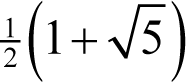can be represented by the tree given in Figure 1.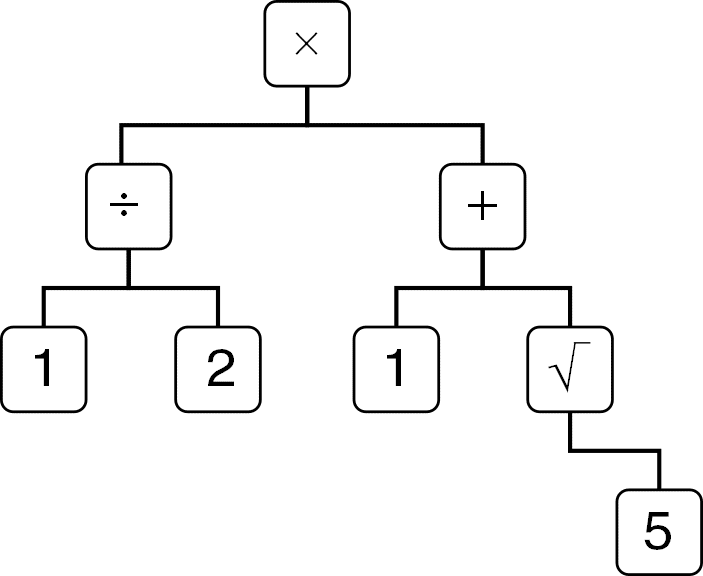Figure 1

The nodes of the tree should be interpreted as the application of the operation they contain to the results of the nodes below it, with the leaf nodes being equated to the numbers they contain.

Such representations are often referred to as expression objects since the values they contain capture complete expressions rather than their results.

## An expression class

We begin with a base class which will represent an abstract expression, as shown in Listing 1.

 ```class expression_object { public: enum{empty=0xE}; virtual ~expression_object() = 0; virtual double approx() const = 0; virtual unsigned char exact(const bignum &n) const = 0; virtual bignum::sign_type sign() const = 0; }; expression_object::~expression_object() { } ``` Listing 1

Naturally, we shall need a virtual destructor to ensure that derived objects are properly cleaned up.

The ``` approx ``` function shall compute the result of the expression using double precision floating point arithmetic whereas the ``` exact ``` method shall return the n th decimal digit of the result of the expression, with negative n being to the right of the decimal point and non-negative n to the left. We shall use the ``` empty ``` constant to indicate that there are no further digits to the left and, in the event that we can exactly represent a number, to the right of the decimal point. Note that we shall not allow leading or trailing zeros for any result other than zero itself, which shall have a zero digit at the zero’th position and shall have all other digits equal to ``` empty ``` .

Finally, the ``` sign ``` function shall indicate the sign of the expression.

The first thing we shall need is a derived class to represent the leaf node constant arbitrary precision integer values. Naturally, we shall use ``` bignum ``` s [ Harris10 ] since we need to be capable of representing any integer, no matter how large. Listing 2 provides the definition of this class.

 ```class integer_expression : public expression_object { public: explicit integer_expression(const bignum &value); virtual ~integer_expression(); virtual double approx() const; virtual unsigned char exact(const bignum &n) const; virtual bignum::sign_type sign() const; const bignum value; }; ``` Listing 2

Note that since we shall treat this as an object type, and hence interact with it through pointers, we can afford to make the member data const and public. We shall deal with assignments by replacing the objects representing expressions rather than changing their states.

The constructor and destructor are straightforward, as shown in Listing 3.

 ```integer_expression::integer_expression( const bignum &value) : value(value) { } integer_expression::~integer_expression() { } ``` Listing 3

The ``` approx ``` member function isn’t so very much harder to implement, provided we don’t care too much about recovering every last digit of precision and are a little blasé about overflow. A naïve implementation is given in Listing 4.

 ```double integer_expression::approx() const { typedef bignum::data_type::const_iterator const_iterator; double x = 0.0; double y = 1.0; size_t i = value.magnitude().size(); const_iterator first = value.magnitude().begin(); const_iterator last = value.magnitude().end(); while(first!=last && x!=y) { const double m = pow(double(bignum::mask)+1.0, double(--i)); y = x; x += m * double(*--last); } return (value.sign()==bignum::positive) ? x : -x; } ``` Listing 4

Here we simply iterate backwards over the digits of the ``` bignum ``` accumulating the sum of their values multiplied by the scale implied by their position.

Note that since the sum of the less significant digits might result in a carry, the true approximate result might require adding 1 to the least significant bit of the mantissa. That said, I'm reasonably happy to trade a relative error of the double precision epsilon in return for such a simple and efficient implementation.

If a call to ``` pow ``` overflows we shall have a result of plus or minus infinity, which isn't so very bad since infinity is a pretty good approximation of a number too big to fit into a ``` double ``` .

The ``` exact ``` member function is even simpler, albeit rather less efficient, provided we have an integer equivalent to the ``` pow ``` function for ``` bignum ``` s, as shown alongside the ``` sign ``` member function in Listing 5.

 ```unsigned char integer_expression::exact(const bignum &n) const { if(n<0L) return empty; const bignum m = pow(bignum(10L), n); if(m>value) return n==0 ? 0 : empty; return (value/m).magnitude().front() % 10; } bignum::sign_type integer_expression::sign() const { return value.sign(); } ``` Listing 5

It would be extremely irritating to interact with this numeric type by directly managing object pointers. To avoid having to do so, we shall use a wrapper class, as shown in Listing 6.

 ```class expression { public: typedef shared_ptr object_type; enum{empty=expression_object::empty}; expression(); expression(const bignum &x); explicit expression(const object_type &x); double approx() const; unsigned char exact(const bignum &n) const; bignum::sign_type sign() const; object_type object() const; int compare(const expression &x) const; expression & negate(); expression & operator+=(const expression &x); expression & operator-=(const expression &x); expression & operator*=(const expression &x); expression & operator/=(const expression &x); private: object_type object_; }; ``` Listing 6

Note that we shall keep track of our expression objects with a reference counted ``` shared_ptr ``` such as the one found in the Boost library.

The constructors are, as has consistently been the case, relatively straightforward as shown in Listing 7.

 ```expression::expression() : object_(new integer_expression(0L)) { } expression::expression(const bignum &x) : object_(new integer_expression(x)) { } expression::expression(const object_type &x) : object_(x ? x : object_type(new integer_expression(0L)) { } ``` Listing 7

Note that, for convenience, we treat uninitialized or null initialised expressions as being equal to 0.

The approximate and exact evaluation functions and the data access method are similarly simple and are given in Listing 8.

 ```double expression::approx() const { assert(object_); return object_->approx(); } unsigned char expression::exact(const bignum &n) const { assert(object_); return object_->exact(n); } bignum::sign expression::sign() const { assert(object_); return object_->sign(); } expression::object_type expression::object() const { return object_; } ``` Listing 8

The ``` compare ``` member function can also be implemented quite simply, provided we are comfortable with the expense of subtracting two expressions during its calculation, as shown in Listing 9.

 ```int expression::compare(const expression &x) const { const expression d = *this - x; if(d.exact(bignum( 0L))==0 && d.exact(bignum( 1L))==empty && d.exact(bignum(-1L))==empty) { return 0; } return d.sign()==bignum::positive ? 1 : -1; } ``` Listing 9

Now, this isn’t going to work until we implement the arithmetic operators, so we shall get right to it!

Unfortunately, these are a little more complicated to get working properly for exact evaluation. We shall, therefore, implement just approximate evaluation for now as an indication of the general approach and we shall return to exact evaluation later.

## Approximate evaluation

We shall use subtraction as an example since we’re already using it; the remaining operators will be more or less the same.

The class definition for the subtraction expression is provided in Listing 10.

 ```class subtraction_expression : public expression_object { public: subtraction_expression(const expression &lhs, const expression &rhs); virtual ~subtraction_expression(); virtual double approx() const; virtual unsigned char exact(const bignum &n) const; virtual bignum::sign_type sign() const; const expression lhs; const expression rhs; }; ``` Listing 10

As ever, we have a trivial constructor and destructor, given in Listing 11.

 ```subtraction_expression::subtraction_expression( const expression &lhs, const expression &rhs) : lhs(lhs), rhs(rhs) { } subtraction_expression::~subtraction_expression() { } ``` Listing 11

The ``` approx ``` function simply approximately evaluates ``` lhs ``` and ``` rhs ``` and subtracts the ``` double ``` resulting from the latter from that resulting from the former, as shown in Listing 12 together with the exact evaluation methods that shall, for now, throw an exception.

 ```double subtraction_expression::approx() const { return lhs.approx() - rhs.approx(); } unsigned char subtraction_expression::exact( const bignum &n) const { throw std::runtime_error(""); return empty; } bignum::sign_type subtraction_expression::sign() const { throw std::runtime_error(""); return bignum::positive; } ``` Listing 12

Note that the return statements aren’t strictly necessary, but they keep my compiler happy.

We use this class in the implementation of the subtraction operation of the expression class, as given in Listing 13.

 ```expression & expression::operator-=(const expression &x) { object_ = object_type( new subtraction_expression(*this, x)); return *this; } ``` Listing 13

Note that we shall effectively use the C++ operator precedence rules to implicitly build the expression tree, saving us from the tedious task of building it explicitly.

For example

`  x+y*z`

will be translated as

`  operator+(x, operator*(y, z))`

which, assuming we implement the free arithmetic operations in terms of the in-place arithmetic operations, would result in

`  expression(x)+=(expression(y)*=z)`

and hence the required expression tree.

## Rearranging expressions

An immediate advantage of such an approach is that by examining the run-time type information of the underlying expression objects we can transform one expression tree into another, simpler one that has an identical value.

For example, we could implement a ``` simplify ``` function that could manipulate the terms in an expression looking for a simpler representation. Using such a function we might expect

`  assert(simplify(x*y/x).object()==y.object());`

to pass for ``` expression ``` s ``` x ``` and ``` y ``` .

## Expression variables

From here it is but a short step to implement an expression object to represent algebraic variables and I should like to explore the ramifications of this before discussing the exact evaluation of expressions. Listing 14 illustrates just such a class.

 ```class variable_expression : public expression_object { public: explicit variable_expression( const expression &value); virtual ~variable_expression(); virtual double approx() const; virtual unsigned char exact(const bignum &n) const; virtual bignum::sign_type sign() const; const expression &value; }; ``` Listing 14

The constructor and destructor are given in Listing 15. Note that we can give the variable a value by assigning to the ``` expression ``` that it holds a reference to.

 ```variable_expression::variable_expression( const expression &value) : value(value) { } variable_expression::~variable_expression() { } ``` Listing 15

The evaluation member functions simply forward to the ``` value ``` reference, as shown in Listing 16.

 ```double variable_expression::approx() const { return value.approx(); } unsigned char variable_expression::exact(const bignum &n) const { return value.exact(n); } bignum::sign_type variable_expression::sign() const { return value.sign(); } ``` Listing 16

## Computer algebra systems

Now that we have an algebraic variable, we can represent algebraic expression and apply ``` simplify ``` to them too. In this sense expression objects lie at the heart of computer algebra systems such as Mathematica, MathCAD and even some top of the range calculators.

Figure 2 illustrates the result of simplifying x × y ÷ x on my own calculator [ Texas ].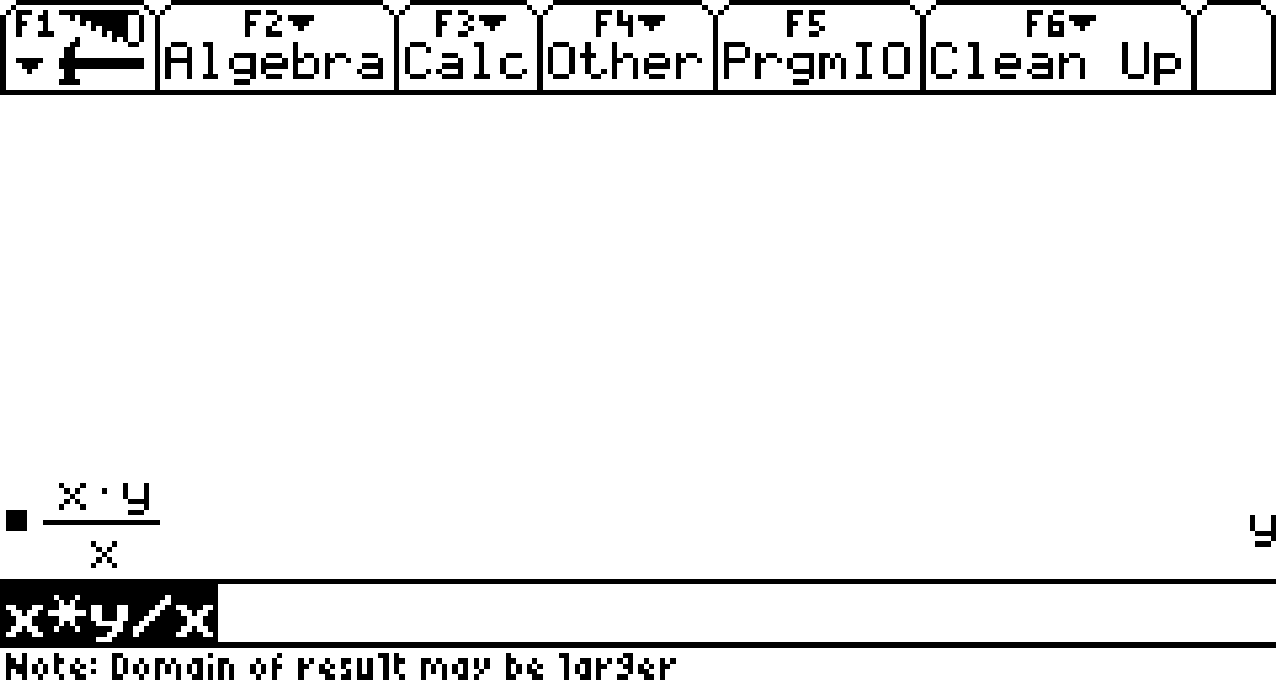Figure 2

We can go further still; if ``` simplify ``` can transform an expression tree than why not ``` differentiate ``` , or ``` integrate ``` or any other algebraic manipulation for that matter?

Figure 3 illustrates the calculation of the derivative of e x at 1 and conclusively demonstrates that computer algebra systems are immune to the problems that arise from cancellation error when approximating it using finite differences.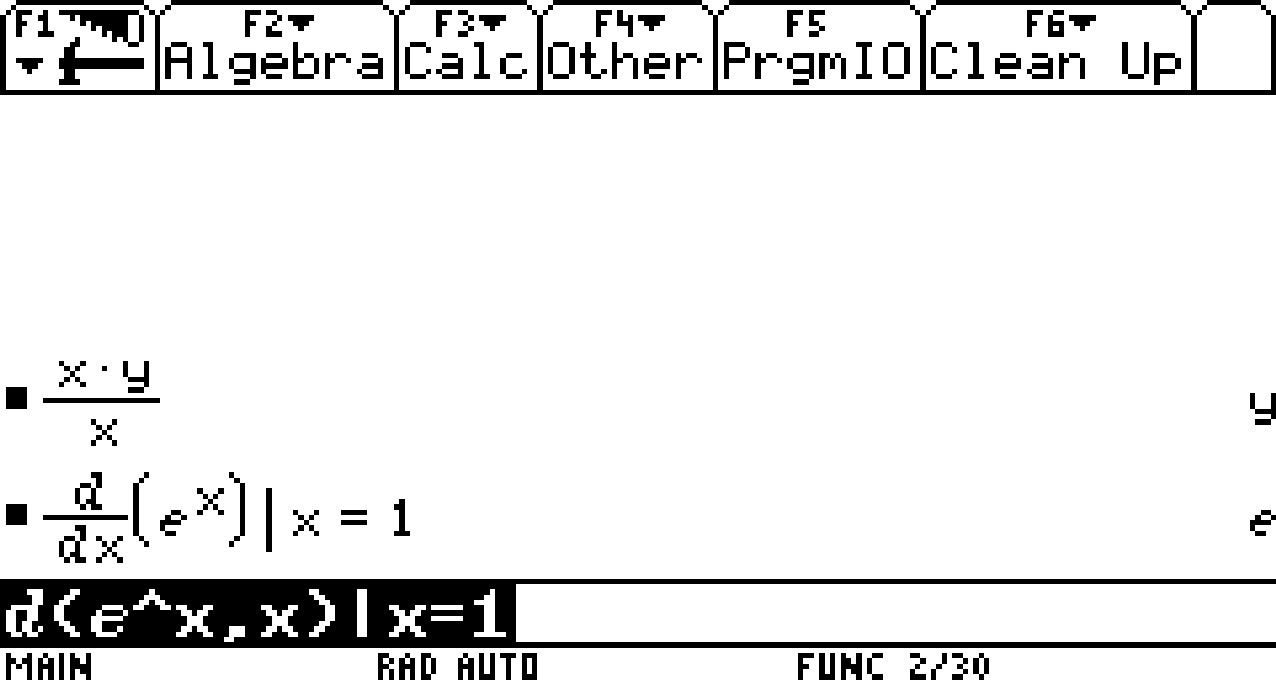Figure 3

Figure 4 illustrates the calculation of the indefinite integral of x ln( x ). This is quite a tricky integral unless you are familiar with the technique of integration by parts, which my calculator evidently is.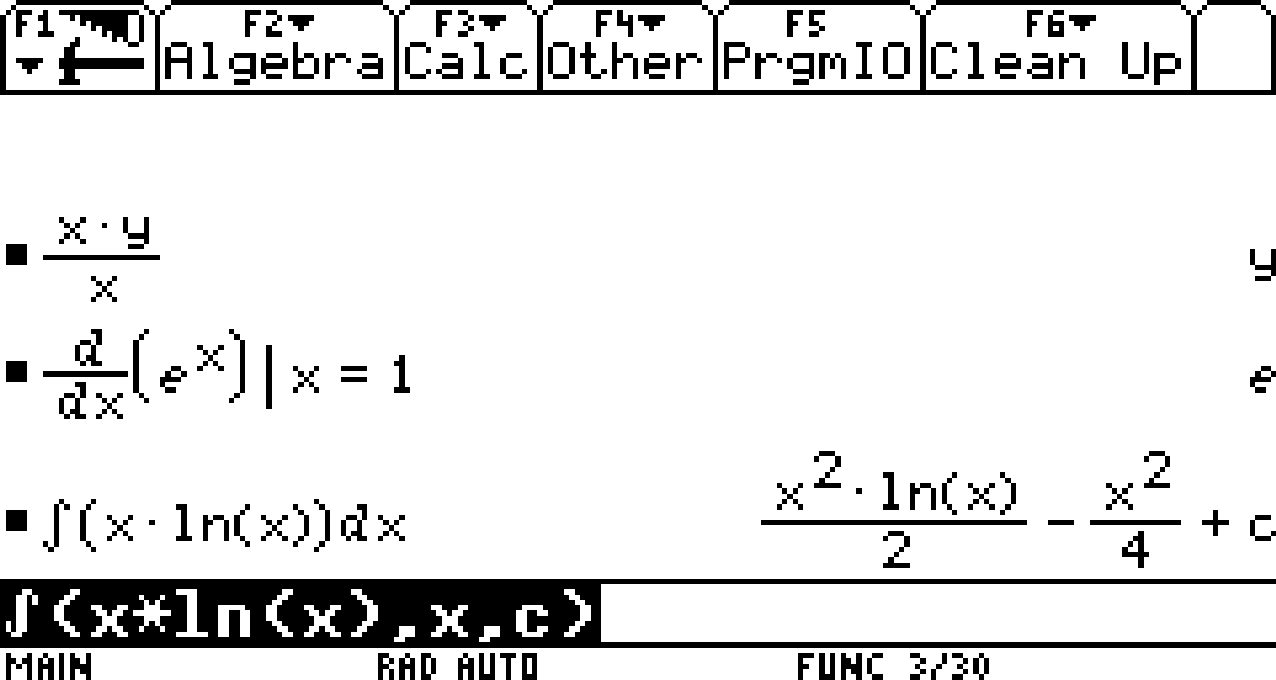Figure 4

To check that this is the correct answer we need only differentiate it and confirm that we get the expression being integrated.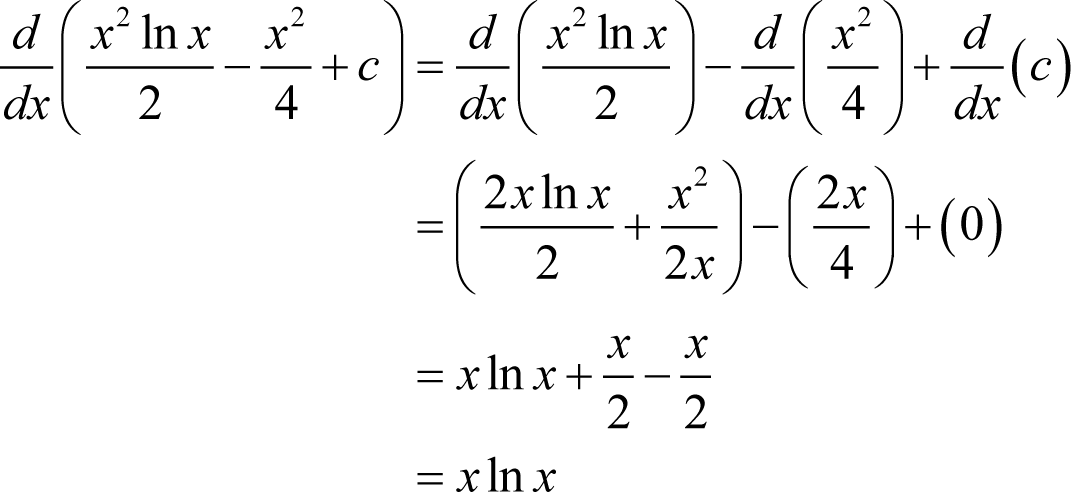Unfortunately, however, closed form results may be impossible to achieve in general. As an example, consider the expression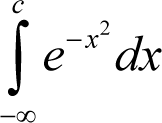My calculator’s evaluation of this reflects the fact that it has no closed form solution, as shown in Figure 5.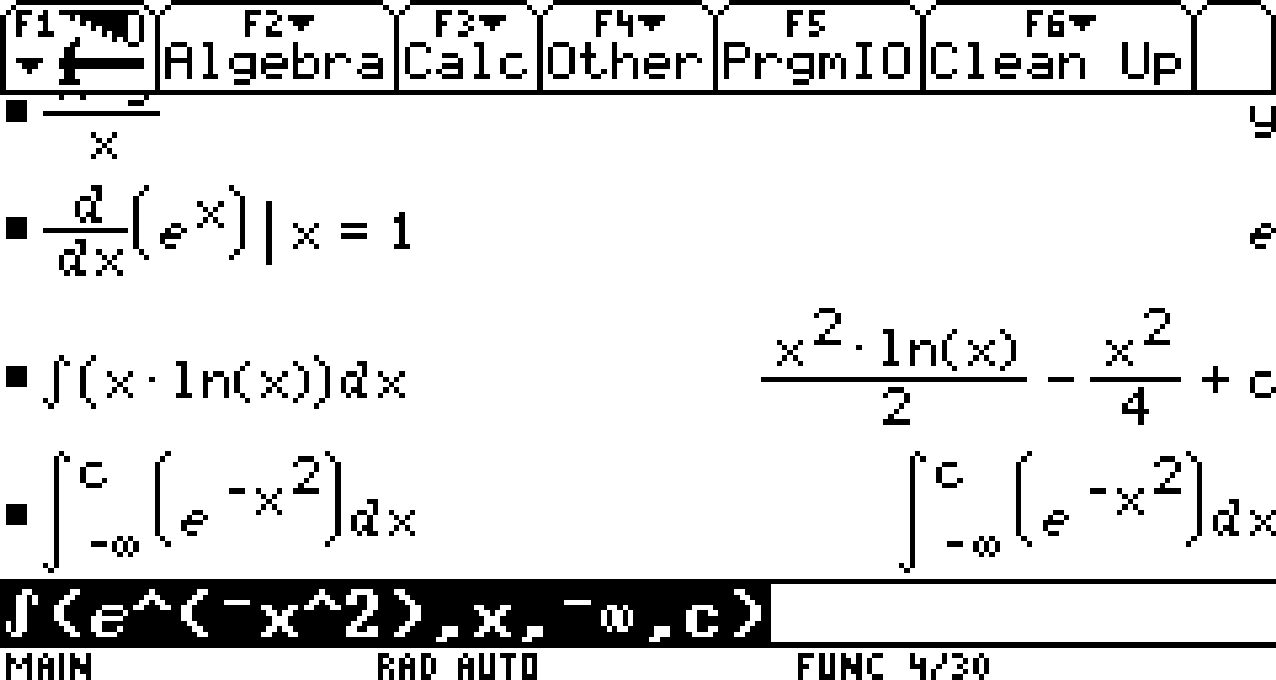Figure 5

In practice computer algebra systems are extremely good at applying lengthy sequences of relatively simple manipulations but tend to struggle when more subtle sequences of transformations are required.

## Exact evaluation

I’m afraid that I must admit that I’m not entirely sure how to do it. Presumably we shall need to implement algorithms that can perform numerical operations to arbitrary precision and cache any working data so that we can extend the number of digits without recalculating those that we have already found.

This might not be too unreasonably difficult for basic arithmetic, but I suspect that implementing numerical algorithms such as integration and differentiation might prove a little trickier. By trickier, I naturally mean demonstrably impossible in general.

Unfortunately there is one major problem that such approaches cannot address. Recall that in order to make comparisons tractable we mandated that exactly representable numbers must have no trailing zeros. If they are not terminated with an ``` empty ``` value we cannot know that we have exhausted every non-zero digit after the decimal point.

For many expressions it is not a simple task to determine if a digit should have an ``` empty ``` value. As an example, consider the expression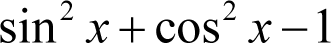This is exactly equal to zero for every value of x , but the first two terms won’t be exactly representable as a decimal fraction for almost all values of x . The addition of the first two terms shall inevitably be trapped in the endless production of zeros, hopelessly searching for a non-zero trailing digit.

The only way we shall escape this fate is to implement a full blown computer algebra system that can simplify all such difficult expressions into forms that can be computed in finite time.

No such system currently exists and, I am sorry to report, no such system ever will.

In 1931 Kurt Gödel proved that there are either infinitely many mathematical propositions that cannot be proven or disproven, or that it is possible to prove propositions that are false and that consequently the rules of mathematics are internally inconsistent [ Gödel31 ].

This caused something of a stir in the mathematical community, who had hitherto been labouring under the illusion that both everything that was true could be proven and that everything that could be proven was true.

Modern mathematicians have come to terms with the fact that there are unprovable truths, or more accurately that there are undecidable propositions, mainly because the alternative is far too bitter a pill to swallow; internal consistency is simply too important to sacrifice.

Alan Turing took up the torch when he settled the decision problem and demonstrated that it wasn’t always possible to determine whether a proposition was decidable or not [ Turing37 ].

Expression objects are therefore superducks; capable of meeting all of our numerical requirements in a single bound but, like their counterpart Superman, are unfortunately wholly fictional in practice.

Quack, quack and away!

[Gödel31] Gödel, K., ‘Über formal unentscheidbare Sätze der Principia Mathematica und verwandter Systeme’, I. Monatshefte für Mathematik und Physik , vol. 38, pp. 173-198, 1931.

[Harris10] Harris, R., ‘You’re Going to Have to Think; Why Fixed Point Won’t Cure Your Floating Point Blues’, Overload 100 , ACCU, 2010

[Texas] Texas Instruments Voyage 200

[Turing37] Turing, A., ‘On Computable Numbers, with an Application to the Entscheidungsproblem’, Proceedings of the London Mathematical Society , Series 2, Vol. 42, pp. 230-265, 1937.